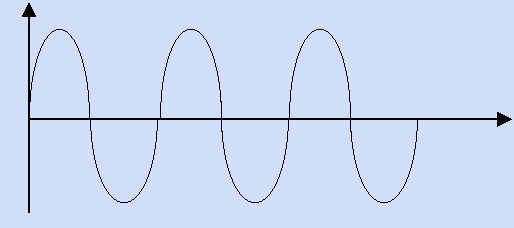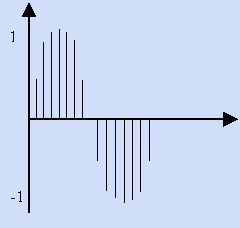### What is DSP?

DSP (Digital Signal Processing) can be described as the manipulation or transformation of a Discrete signal by means of arithmetic and mathematical formulae. But there are 2 classifications for audio signals to be considered:

1.  Discrete
2. Continuous

Continuous signals can be described by analysing the analogue synthesizer and its oscillator outputs. The circuitry involved to generate such signals consists of 2 elements of control, signal amplitude (volume) and signal frequency (pitch). These have been implemented with additional analogue circuitry to enable voltage control of the elements. i.e. Voltage Controlled Oscillators (VCO’s) and Voltage Controlled Amplifiers (VCA’s), where the voltage applied at the input of the VCA is directly proportional to the level of signal amplification and the voltage applied to the input of the VCO is directly proportional to the frequency that it generates.

By considering the function of the VCO in greater detail, it is possible to understand the distinction between Continuous signals and Discrete signals. Once the VCO is 'triggered' by a real voltage at the input the circuitry begins to oscillate, and the output signal performs a cycle. The cycle characterises signal 'tone' or 'timbre', but the frequency by which the cycle repeats every second will determine the pitch of it. The cycle type has been available on most early analogue synthesizers as the following wave types:

•  Square
• Triangle
• Saw
•  Pulse

... and the most basic of all is the

•  Sine wave, derived from the trigonometric function for a sinusoid.

For analogue oscillators, there are infinite possibilities to determine a particular cycle/period regardless of the duration of it in terms of time. This characterises the signal as a Continuous signal.

A Discrete signal on the other hand does not have infinite possibilities. It is governed by two factors, Sampling rate and Sampling resolution. Sampling rate is defined as the number of samples collected at regular intervals to represent a signal over one second. Figure 1.1a shows a Continuous sine wave generated by an analogue synthesizer, and Figure 1.1b shows a close up of a single period of the same sine wave, that has been sampled, i.e. converted into a Discrete - time - signal.Fig 1.1aFig 1.1b

For a conventional 44.1 kHz digital audio device like a CD player, the Discrete time step can be evaluated as 1/44100, which means that the CD player provides a number at the output every 22.7micro seconds (1/44100). This device will store 44100 16-bit numbers every second for one channel (i.e.. left or right) of sound.This brings us to the argument concerning the second factor that governs digital audio processing; Sampling Resolution. As discussed previously a signal or cycle can be simplified into frequency (pitch) and amplitude (volume). Discrete signal processing states that the maximum and minimum amplitudes of a signal have to be represented by a finite number of values, and hence can be incorporated into a digital system. The CD uses 65536 values to determine the amplitude of the signal being converted from digital to analogue. This number can be represented in many different ways, but here are just a few and you can work the rest out for yourself... (For more advanced info, see the article that describes Psycho Acoustic Resolution Standards. Perceptive coding has utilised the 'real' response of the human ear to enable compression of audio data flow, but professional digital signal processing architectures can utilise this principle inversely to produce higher quality audio data @ the same resolution.)

1.  65536
2.  2 to the power 16
3.  16 - bit number
4.  word
5.  short int
6.  2 bytes
7.  2 characters (2 x 8-bit ASCII characters on a computer system)
8.  4 nibbles
9.  96 dB

We can now construct one of the most important equations for evaluating the art of DSP, and later the reader will realise how important it actually is for time, cost, and quality of the system you are designing:

The equation describes the data flow of the digital system being implemented, thus, for CD quality:

bytes / second = SRate x C x SRes = 44100 x 2 x 2(bytes) = 176400 bytes/sec

This can be re-written to describe the data flow in bits (see list above), thus:

bits / second = SRate x C x SRes = 44100 x 2 x 16(bits) = 1.4112 mega bits/sec